# Stage Storage Calculations

Stage Storage calculations use the procedures below based on the storage type selected.

## Elevation Contours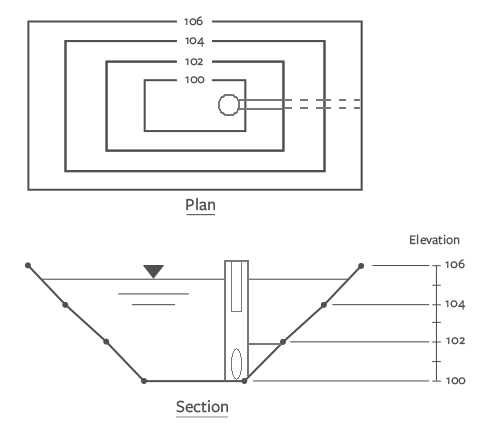Hydrology Studio uses either the average-end-area method applied vertically or the Conic method. The Conic method uses this equation: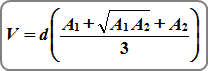Where:
V = storage
d = change in elevation between points 1 and 2
A1 = surface area at elevation 1
A2 = surface area at elevation 2

## TrapezoidTrapezoidal shaped ponds are computed by: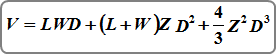Where:

V = storage volume at stage D
D = stage or depth
L = bottom length
W = bottom width
Z = side slope, (Z:1) (horizontal to 1-vertical)

## Generic Underground Chambers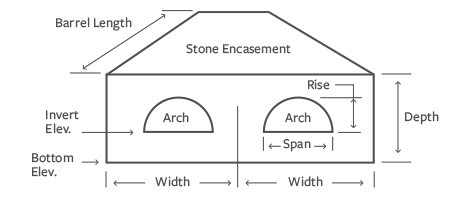Volume of a generic chamber pipe only is computed by: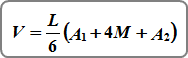Where:
V = storage volume
L = pipe length
A1 = cross-sectional area of depth at downstream end
A2 = cross-sectional area of depth at upstream end
M = cross-sectional area of depth at midsection

When the pipe slope equals zero, Volume = L x A1

It should be noted that the top of the stone encasement is not subject to an inputted barrel slope. Instead, the software assumes the encasement is level on top but sloped on the bottom. This will tend to increase the total encasement depth at the downstream end (Depth as inputted plus L x Slope) and resulting storage.